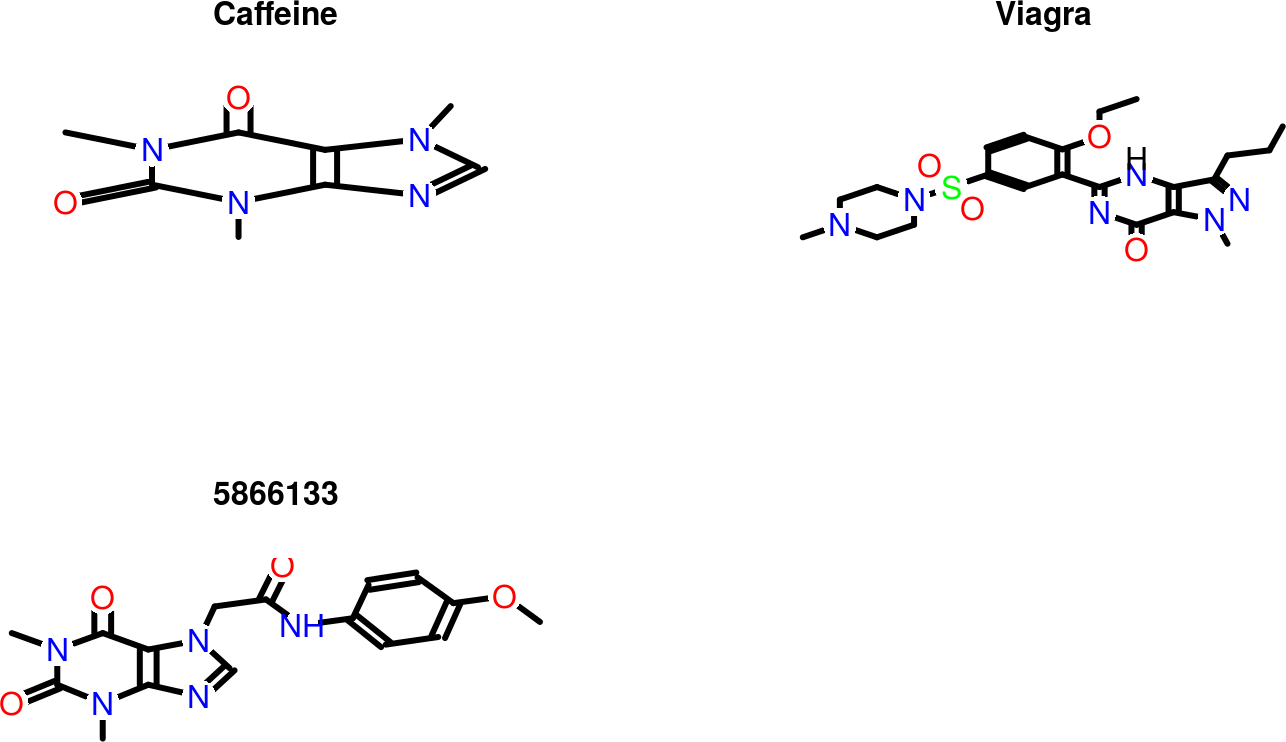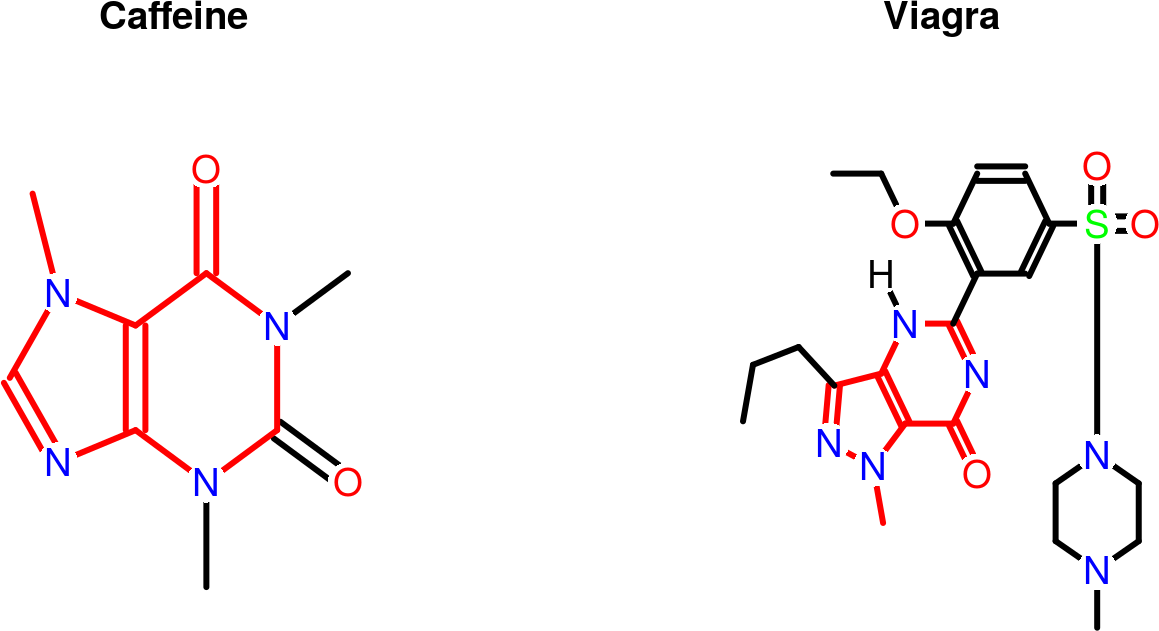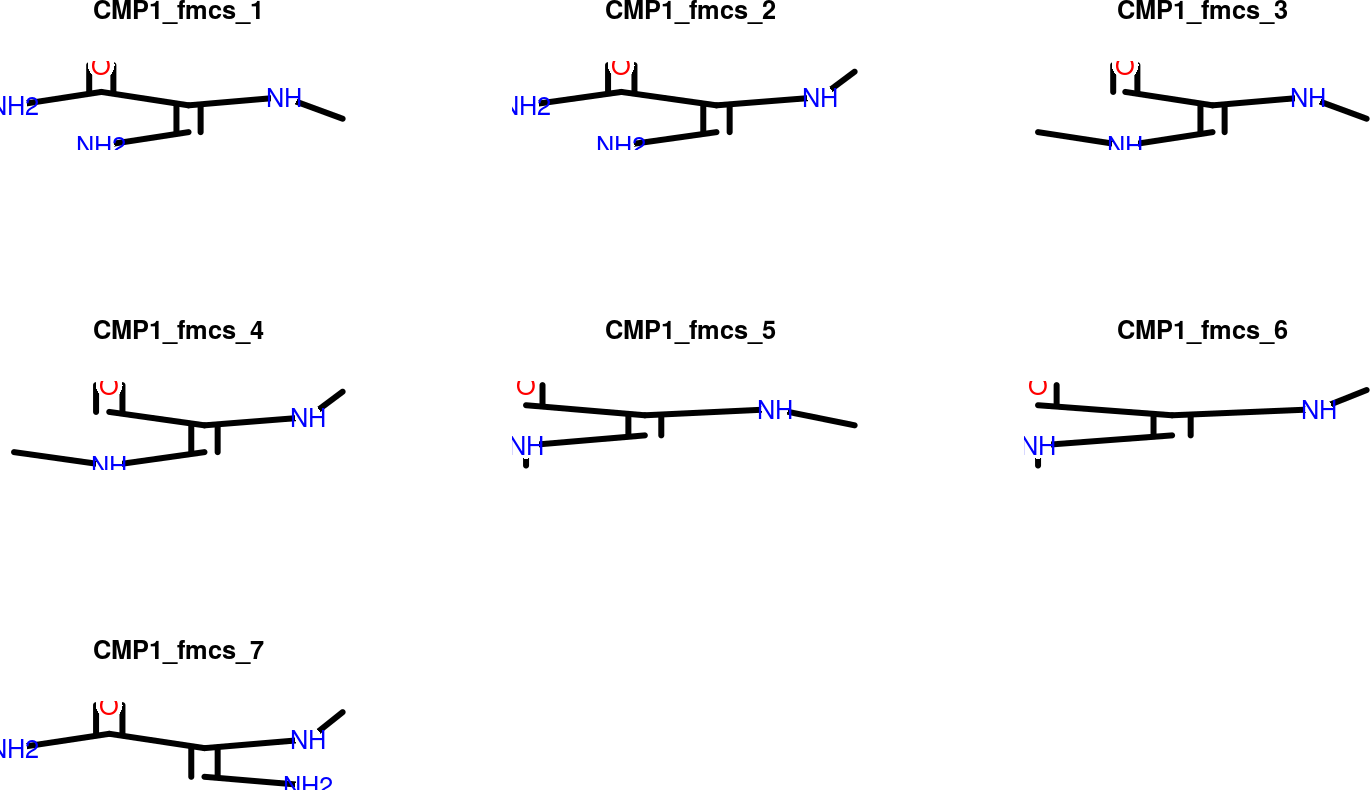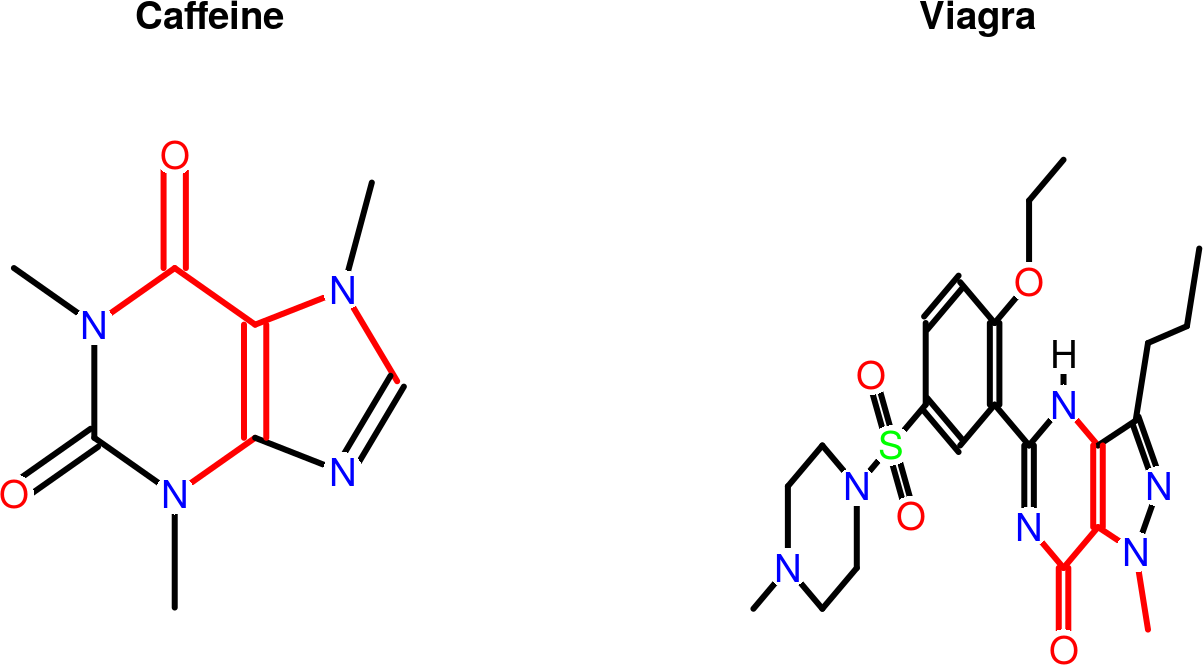# Contents

Note: the most recent version of this tutorial can be found here and a short overview slide show here.

# 1 Introduction

Maximum common substructure (MCS) algorithms rank among the most sensitive and accurate methods for measuring structural similarities among small molecules. This utility is critical for many research areas in drug discovery and chemical genomics. The MCS problem is a graph-based similarity concept that is defined as the largest substructure (sub-graph) shared among two compounds (Wang et al. 2013; Cao, Jiang, and Girke 2008). It fundamentally differs from the structural descriptor-based strategies like fingerprints or structural keys. Another strength of the MCS approach is the identification of the actual MCS that can be mapped back to the source compounds in order to pinpoint the common and unique features in their structures. This output is often more intuitive to interpret and chemically more meaningful than the purely numeric information returned by descriptor-based approaches. Because the MCS problem is NP-complete, an efficient algorithm is essential to minimize the compute time of its extremely complex search process. The `fmcsR` package implements an efficient backtracking algorithm that introduces a new flexible MCS (FMCS) matching strategy to identify MCSs among compounds containing atom and/or bond mismatches. In contrast to this, other MCS algorithms find only exact MCSs that are perfectly contained in two molecules. The details about the FMCS algorithm are described in the Supplementary Materials Section of the associated publication (Wang et al. 2013). The package provides several utilities to use the FMCS algorithm for pairwise compound comparisons, structure similarity searching and clustering. To maximize performance, the time consuming computational steps of `fmcsR` are implemented in C++. Integration with the `ChemmineR` package provides visualization functionalities of MCSs and consistent structure and substructure data handling routines (Cao et al. 2008; Backman, Cao, and Girke 2011). The following gives an overview of the most important functionalities provided by `fmcsR`.

# 2 Installation

The R software for running `fmcsR` and `ChemmineR` can be downloaded from CRAN (http://cran.at.r-project.org/). The `fmcsR` package can be installed from an open R session using the `biocLite` install command.

``````source("http://bioconductor.org/biocLite.R")
biocLite("fmcsR") ``````

# 3 Quick Overview

To demo the main functionality of the `fmcsR` package, one can load its sample data stored as `SDFset` object. The generic `plot` function can be used to visualize the corresponding structures.

``````library(fmcsR)
data(fmcstest)
plot(fmcstest[1:3], print=FALSE) ``````The `fmcs` function computes the MCS/FMCS shared among two compounds, which can be highlighted in their structure with the `plotMCS` function.

``````test <- fmcs(fmcstest, fmcstest, au=2, bu=1)
plotMCS(test,regenCoords=TRUE) ``````# 4 Documentation

``library("fmcsR") # Loads the package ``
``````library(help="fmcsR") # Lists functions/classes provided by fmcsR
library(help="ChemmineR") # Lists functions/classes from ChemmineR
vignette("fmcsR") # Opens this PDF manual
vignette("ChemmineR") # Opens ChemmineR PDF manual ``````

The help documents for the different functions and container classes can be accessed with the standard R help syntax.

``````?fmcs
?"MCS-class"
?"SDFset-class" ``````

# 5 MCS of Two Compounds

## 5.1 Data Import

The following loads the sample data set provided by the `fmcsR` package. It contains the SD file (SDF) of 3 molecules stored in an `SDFset` object.

``````data(fmcstest)
sdfset <- fmcstest
sdfset ``````
``## An instance of "SDFset" with 3 molecules``

Custom compound data sets can be imported and exported with the `read.SDFset` and `write.SDF` functions, respectively. The following demonstrates this by exporting the `sdfset` object to a file named `sdfset.sdf`. The latter is then reimported into R with the `read.SDFset` function.

``````write.SDF(sdfset, file="sdfset.sdf")

## 5.2 Compute MCS

The `fmcs` function accepts as input two molecules provided as `SDF` or `SDFset` objects. Its output is an S4 object of class `MCS`. The default printing behavior summarizes the MCS result by providing the number of MCSs it found, the total number of atoms in the query compound \(a\), the total number of atoms in the target compound \(b\), the number of atoms in their MCS \(c\) and the corresponding Tanimoto Coefficient. The latter is a widely used similarity measure that is defined here as \(c/(a+b-c)\). In addition, the Overlap Coefficient is provided, which is defined as \(c/min(a,b)\). This coefficient is often useful for detecting similarities among compounds with large size differences.

``````mcsa <- fmcs(sdfset[], sdfset[])
mcsa ``````
``````## An instance of "MCS"
##  Number of MCSs: 7
##  CMP1: 14 atoms
##  CMP2: 33 atoms
##  MCS: 8 atoms
##  Tanimoto Coefficient: 0.20513
##  Overlap Coefficient: 0.57143``````
``````mcsb <- fmcs(sdfset[], sdfset[])
mcsb ``````
``````## An instance of "MCS"
##  Number of MCSs: 1
##  CMP1: 14 atoms
##  CMP2: 25 atoms
##  MCS: 14 atoms
##  Tanimoto Coefficient: 0.56
##  Overlap Coefficient: 1``````

If `fmcs` is run with `fast=TRUE` then it returns the numeric summary information in a named `vector`.

``fmcs(sdfset, sdfset, fast=TRUE)``
``````##           Query_Size          Target_Size             MCS_Size Tanimoto_Coefficient
##           14.0000000           33.0000000            8.0000000            0.2051282
##  Overlap_Coefficient
##            0.5714286``````

## 5.3 Class Usage

The `MCS` class contains three components named `stats`, `mcs1` and `mcs2`. The `stats` slot stores the numeric summary information, while the structural MCS information for the query and target structures is stored in the `mcs1` and `mcs2` slots, respectively. The latter two slots each contain a `list` with two subcomponents: the original query/target structures as `SDFset` objects as well as one or more numeric index vector(s) specifying the MCS information in form of the row positions in the atom block of the corresponding `SDFset`. A call to `fmcs` will often return several index vectors. In those cases the algorithm has identified alternative MCSs of equal size.

``slotNames(mcsa) ``
``##  "stats" "mcs1"  "mcs2"``

Accessor methods are provided to return the different data components of the `MCS` class.

``stats(mcsa) # or mcsa[["stats"]] ``
``````##           Query_Size          Target_Size             MCS_Size Tanimoto_Coefficient
##           14.0000000           33.0000000            8.0000000            0.2051282
##  Overlap_Coefficient
##            0.5714286``````
``````mcsa1 <- mcs1(mcsa) # or mcsa[["mcs1"]]
mcsa2 <- mcs2(mcsa) # or mcsa[["mcs2"]]
mcsa1 # returns SDFset component``````
``````## \$query
## An instance of "SDFset" with 1 molecules``````
``mcsa1[][1:2] # return first two index vectors ``
``````## \$CMP1_fmcs_1
##   3  8  7  4  9  5 11  1
##
## \$CMP1_fmcs_2
##   3  8  7  4  9  5  1 13``````

The `mcs2sdfset` function can be used to return the substructures stored in an `MCS` instance as `SDFset` object. If `type='new'` new atom numbers will be assigned to the subsetted SDF, while `type='old'` will maintain the atom numbers from its source. For details consult the help documents `?mcs2sdfset` and `?atomsubset`.

``````mcstosdfset <- mcs2sdfset(mcsa, type="new")
plot(mcstosdfset[], print=FALSE) ``````To construct an `MCS` object manually, one can provide the required data components in a `list`.

``````mylist <- list(stats=stats(mcsa), mcs1=mcs1(mcsa), mcs2=mcs2(mcsa))
as(mylist, "MCS") ``````
``````## An instance of "MCS"
##  Number of MCSs: 7
##  CMP1: 14 atoms
##  CMP2: 33 atoms
##  MCS: 8 atoms
##  Tanimoto Coefficient: 0.20513
##  Overlap Coefficient: 0.57143``````

# 6 FMCS of Two Compounds

If `fmcs` is run with its default paramenters then it returns the MCS of two compounds, because the mismatch parameters are all set to zero. To identify FMCSs, one has to increase the number of upper bound atom mismatches `au` and/or bond mismatches `bu` to interger values above zero.

``plotMCS(fmcs(sdfset, sdfset, au=0, bu=0)) ````plotMCS(fmcs(sdfset, sdfset, au=1, bu=1)) ``